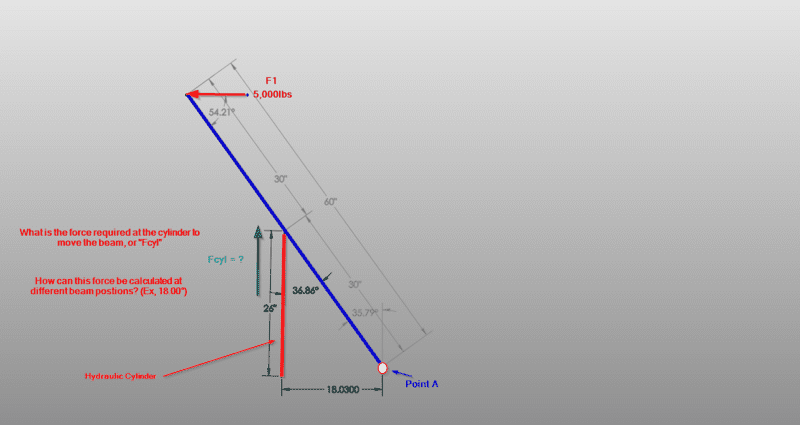# Cylinder Force Calculations

• Tmuell
In summary, the conversation is about calculating the force required to lift and rotate a beam. The beam has a 5,000lb force pushing to the left and the person is trying to size a cylinder to move the load back and forth. The application is designing an arm to move a material across a frame and there are concerns about the safety and accuracy of the given information. The person is advised to review free body diagrams and consider the horizontal forces involved in order to accurately determine the required force for the cylinder.

#### Tmuell

TL;DR Summary
Looking to calculate required force output from the cylinder the lift the beam with the acting load at different positions.I am trying to calculate the force required to lift/move the beam clockwise from the shown position. I have a force (F1) acting at the end of the beam, 5,000lbs pushing to the left, as shown. What is "Fcyl", or the force required by the cylinder to life the beam with the acting load, and rotate about the beams fixed point, point A.

Welcome to PF. What is the application?

berkeman said:
Welcome to PF. What is the application?
Thanks. Designing an arm to move a material (load "F1") back and forth across a frame - I need to size a cylinder capable of moving the load to each side about the arm/beams axis.

One of the rules at PF is that we do not allow discussions of dangerous things. A 5000 lb force at the end of a 60" beam creates suspicion that something dangerous is involved. We need more information about the total application in order to decide if we should answer you.

Your profile states that you have a degree in Ag Engineering. Review free body diagrams, especially the part about sum of moments equals zero.

•berkeman
Another thing. Are you sure you have a 5000 lb load acting horizontally? You state that you are "moving a load" left and right. If you are moving a load that weighs 5000 lbf, is not necessarily the same thing as having a load of 5000 lbf horizontally. You will have some resistance to from motion from whatever that load is resting on, maybe its sliding on something smooth, maybe it's on rollers. In order to get a 5000 lbf load horizontally, you are going to have a lot of friction, and/or relatively high acceleration in the horizontal direction.

You have to consider in the horizontal direction:

##\sum \mathbf F = m \mathbf a##